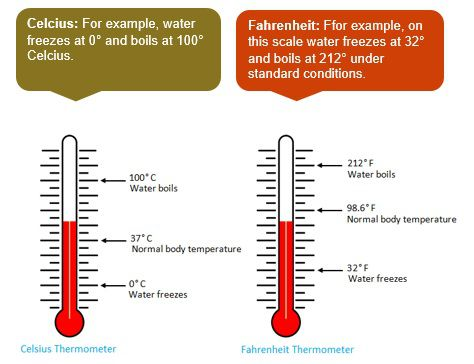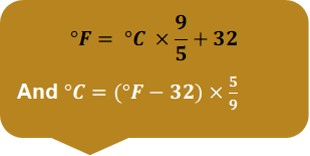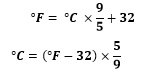Measurement – Temperature

Temperature is defined to check how much the object is hot or cold.

There are two units to define the temperature:For normal body, temperature is = 37°C = 98.6°F

Where, C = Celsius and F = Fahrenheit

Relation between Celsius and Fahrenheit:

This formula will be used in most of the questions of measurement of temperature where we need to convert C into F or vice versa.Example 1: Convert 20°C to Fahrenheit.

Solution: We know that,

Therefore, Temperature in °F =  = 36 + 32 = 68°F

Example 2: Convert 37°F into Celsius.

Solution: We know that,

Therefore  = 2.78°C

Exercise

Question1: Convert the following temperature given in Celsius scale into Fahrenheit scale

1. 25°C
2. 0°C
• 3°C
1. 65°C
2. 20°C

Answer: i) 77°F  ii) 32°F   iii) 104.54°F   iv) 149°F   v) 68°F

Question 2:  Convert the following temperature given in Fahrenheit scale into Celsius scale

1. 200°F
2. 175°F
• 185°F
1. 158°F
2. 68°F

Answers: i) 93.33°C  ii) 79.44°C  iii) 85°C  iv) 70°C  v) 20°C

Recap

• There are two scales to measure temperature i.e. Celsius and Fahrenheit.
• The normal body temperature is 98.4°F or 37°C
• The device used to check body temperature is called thermometer.
• Important Formula: